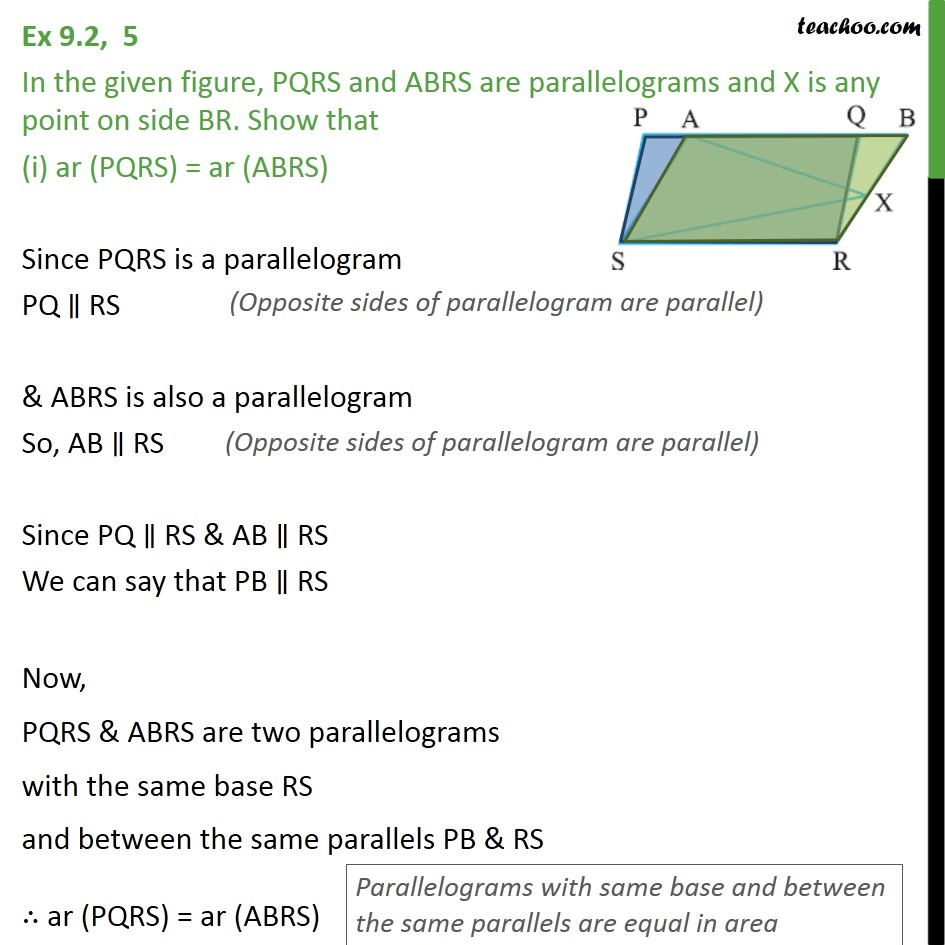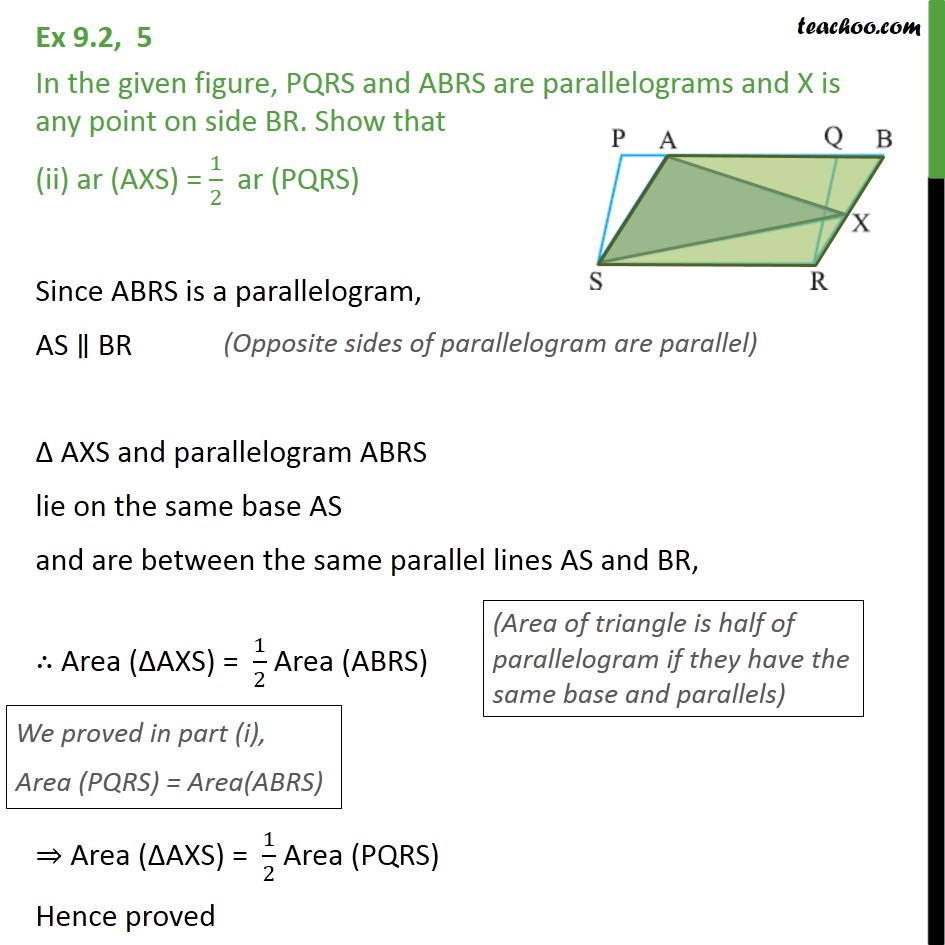Ex 9.2

Chapter 9 Class 9 - Areas of Parallelograms and Triangles [Deleted]
Serial order wiseGet live Maths 1-on-1 Classs - Class 6 to 12

### Transcript

Ex 9.2, 5 In the given figure, PQRS and ABRS are parallelograms and X is any point on side BR. Show that (i) ar (PQRS) = ar (ABRS) Since PQRS is a parallelogram PQ ∥ RS & ABRS is also a parallelogram So, AB ∥ RS Since PQ ∥ RS & AB ∥ RS We can say that PB ∥ RS Now, PQRS & ABRS are two parallelograms with the same base RS and between the same parallels PB & RS ∴ ar (PQRS) = ar (ABRS) Ex 9.2, 5 In the given figure, PQRS and ABRS are parallelograms and X is any point on side BR. Show that (ii) ar (AXS) = 1/2 ar (PQRS) Since ABRS is a parallelogram, AS ∥ BR Δ AXS and parallelogram ABRS lie on the same base AS and are between the same parallel lines AS and BR, ∴ Area (ΔAXS) = 1/2 Area (ABRS) ⇒ Area (ΔAXS) = 1/2 Area (PQRS) Hence proved